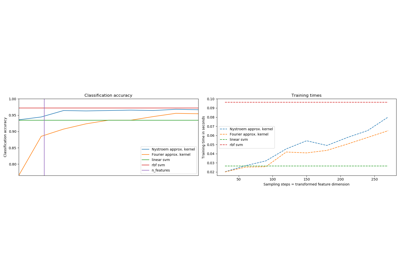# sklearn.kernel_approximation.Nystroem¶

class sklearn.kernel_approximation.Nystroem(kernel='rbf', gamma=None, coef0=None, degree=None, kernel_params=None, n_components=100, random_state=None)[source]

Approximate a kernel map using a subset of the training data.

Constructs an approximate feature map for an arbitrary kernel using a subset of the data as basis.

Read more in the User Guide.

New in version 0.13.

Parameters
kernelstring or callable, default=”rbf”

Kernel map to be approximated. A callable should accept two arguments and the keyword arguments passed to this object as kernel_params, and should return a floating point number.

gammafloat, default=None

Gamma parameter for the RBF, laplacian, polynomial, exponential chi2 and sigmoid kernels. Interpretation of the default value is left to the kernel; see the documentation for sklearn.metrics.pairwise. Ignored by other kernels.

coef0float, default=None

Zero coefficient for polynomial and sigmoid kernels. Ignored by other kernels.

degreefloat, default=None

Degree of the polynomial kernel. Ignored by other kernels.

kernel_paramsmapping of string to any, optional

Additional parameters (keyword arguments) for kernel function passed as callable object.

n_componentsint

Number of features to construct. How many data points will be used to construct the mapping.

random_stateint, RandomState instance or None, optional (default=None)

If int, random_state is the seed used by the random number generator; If RandomState instance, random_state is the random number generator; If None, the random number generator is the RandomState instance used by np.random.

Attributes
components_array, shape (n_components, n_features)

Subset of training points used to construct the feature map.

component_indices_array, shape (n_components)

Indices of components_ in the training set.

normalization_array, shape (n_components, n_components)

Normalization matrix needed for embedding. Square root of the kernel matrix on components_.

RBFSampler

An approximation to the RBF kernel using random Fourier features.

sklearn.metrics.pairwise.kernel_metrics

List of built-in kernels.

References

• Williams, C.K.I. and Seeger, M. “Using the Nystroem method to speed up kernel machines”, Advances in neural information processing systems 2001

• T. Yang, Y. Li, M. Mahdavi, R. Jin and Z. Zhou “Nystroem Method vs Random Fourier Features: A Theoretical and Empirical Comparison”, Advances in Neural Information Processing Systems 2012

Examples

>>> from sklearn import datasets, svm
>>> from sklearn.kernel_approximation import Nystroem
>>> X, y = datasets.load_digits(n_class=9, return_X_y=True)
>>> data = X / 16.
>>> clf = svm.LinearSVC()
>>> feature_map_nystroem = Nystroem(gamma=.2,
...                                 random_state=1,
...                                 n_components=300)
>>> data_transformed = feature_map_nystroem.fit_transform(data)
>>> clf.fit(data_transformed, y)
LinearSVC()
>>> clf.score(data_transformed, y)
0.9987...


Methods

 fit(self, X[, y]) Fit estimator to data. fit_transform(self, X[, y]) Fit to data, then transform it. get_params(self[, deep]) Get parameters for this estimator. set_params(self, \*\*params) Set the parameters of this estimator. transform(self, X) Apply feature map to X.
__init__(self, kernel='rbf', gamma=None, coef0=None, degree=None, kernel_params=None, n_components=100, random_state=None)[source]

Initialize self. See help(type(self)) for accurate signature.

fit(self, X, y=None)[source]

Fit estimator to data.

Samples a subset of training points, computes kernel on these and computes normalization matrix.

Parameters
Xarray-like of shape (n_samples, n_features)

Training data.

fit_transform(self, X, y=None, **fit_params)[source]

Fit to data, then transform it.

Fits transformer to X and y with optional parameters fit_params and returns a transformed version of X.

Parameters
Xnumpy array of shape [n_samples, n_features]

Training set.

ynumpy array of shape [n_samples]

Target values.

**fit_paramsdict

Returns
X_newnumpy array of shape [n_samples, n_features_new]

Transformed array.

get_params(self, deep=True)[source]

Get parameters for this estimator.

Parameters
deepbool, default=True

If True, will return the parameters for this estimator and contained subobjects that are estimators.

Returns
paramsmapping of string to any

Parameter names mapped to their values.

set_params(self, **params)[source]

Set the parameters of this estimator.

The method works on simple estimators as well as on nested objects (such as pipelines). The latter have parameters of the form <component>__<parameter> so that it’s possible to update each component of a nested object.

Parameters
**paramsdict

Estimator parameters.

Returns
selfobject

Estimator instance.

transform(self, X)[source]

Apply feature map to X.

Computes an approximate feature map using the kernel between some training points and X.

Parameters
Xarray-like of shape (n_samples, n_features)

Data to transform.

Returns
X_transformedarray, shape=(n_samples, n_components)

Transformed data.

## Examples using sklearn.kernel_approximation.Nystroem¶